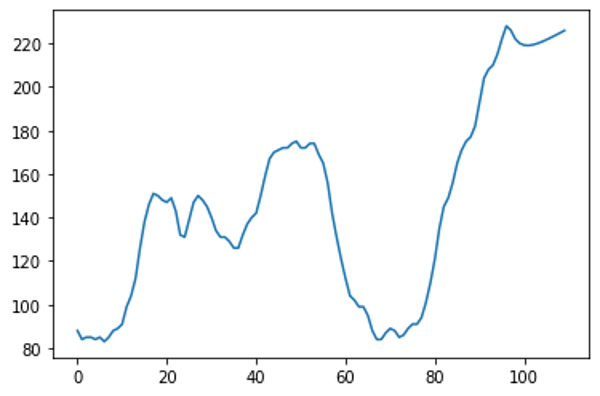Related Tags

python

# What is ARIMA in Python?

## What is ARIMA?

The ARIMA modelstands for AutoRegressive Integrated Moving Average allows us to forecast a time series using the series’ past values.

A time series is a collection of data points collected at constant time intervals. Time series are used to forecast future values based on previous values.

A stationary time series is one whose statistical properties (mean, variance, autocorrelation, etc.) are all constant over time. A non-stationary series is one whose statistical properties change over time.

## ARIMA model

An ARIMA model is characterized by three terms: p, d, q.

• p is the order of the ARauto-regressive part of the model term.

• q is the order of the MAmoving average term.

• d is the number of differencingthe number of past time points to subtract from the current value required to make the time series stationary.

## ARIMA model in python

In this example, we will predict the next 10 days of stock prices from a given data of 100 days.

## Step 1

Import the relevant libraries to perform time series forecasting:

import numpy as np, pandas as pd
import statsmodels.tsa.stattools as ts
from statsmodels.tsa.arima_model import ARIMA
import matplotlib.pyplot as plt


## Step 2

Upload the relevant dataset using pandas.read_csv() method:

file = pd.read_csv("data.csv")
// prices is a field in .csv file containing all stock prices.
stock_price = df['prices']


You can view this data in stock_price using the plt.plot() method:

plt.plot(stock_price)


Below is the code to an output the variation in stock price for the last 100 days. It also contains a .csv file with sample stock prices.

## Step 3

Initialize the ARIMA model and set the values of p, d, and q as 1, 1, and 2.

model = ARIMA(stock_price, order=(1,1,2))
model_fit = model.fit(disp=0)
// summary provides a detailed summary of the time series model
print(model_fit.summary())


## Step 4

Let’s predict the next 10 values and plot them on a graph:

pred = model_fit.predict(100,109,typ='levels')
// 100-109, refers to the next 10 values after the value at 99th index.
newarr = []
for i in price:
newarr.append(i)

for x in pred:
newarr.append(x)

plt.plot(newarr)## Complete code

main.py
data.csv
import numpy as np, pandas as pd
import matplotlib.pyplot as plt
import statsmodels.tsa.stattools as ts
from statsmodels.tsa.arima_model import ARIMA

# prices is a field in .csv file containing all stock prices.
stock_price = df['prices']
plt.plot(stock_price)

# ARIMA model
model = ARIMA(stock_price, order=(1,1,2))
model_fit = model.fit(disp=0)
# summary provides a detailed summary of the time series model
print(model_fit.summary())

# Predicting values
pred = model_fit.predict(100,109,typ='levels')
# 100-109, refers to the next 10 values after the value at 99th index.

# newarr array combines the predicted the stock values in one array
newarr = []
for i in price:
newarr.append(i)

for x in pred:
newarr.append(x)

plt.plot(newarr)


RELATED TAGS

python

CONTRIBUTOR